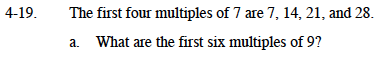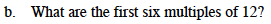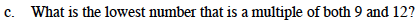### Home > MC1 > Chapter 4 > Lesson 4.1.2 > Problem4-19

4-19.
1. . The first four multiples of 7 are 7, 14, 21, and 28. Homework Help ✎

1. What are the first six multiples of 9?

2. What are the first six multiples of 12?

3. What is the lowest number that is a multiple of both 9 and 12?The first multiple of 9 is 9(1) = 9.
The second multiple of 9 is 9(2) = 18.
The third multiple of 9 is 9(3) = ?Look to part (a) for assistance.This is the first number that appears in both of your lists.